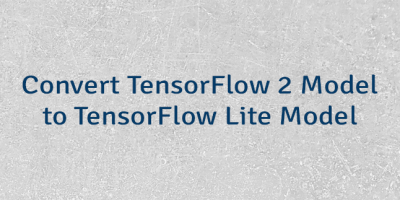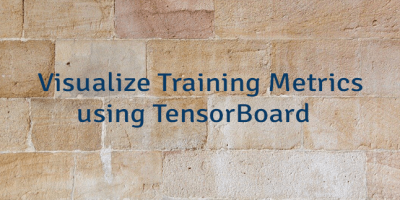## Classify Images using MobileNet and TensorFlow Lite

MobileNet is a convolutional neural network (CNN) that designed for mobile and embedded devices. MobileNet is often used for image classification. This tutorial demonstrates how to classify images using MobileNet...## Convert TensorFlow 2 Model to TensorFlow Lite Model

TensorFlow 2 provides the TFLiteConverter which allows converting a TensorFlow 2 model to TensorFlow Lite model. This model uses the .tflite file extension. TensorFlow Lite models can be executed using...## Visualize Training Metrics using TensorBoard

TensorBoard is a tool which allows visualizing training metrics (e.g. loss and accuracy), model graph, activation histograms, profiling results, etc. This tutorial demonstrates how to visualize training metrics using TensorBoard...